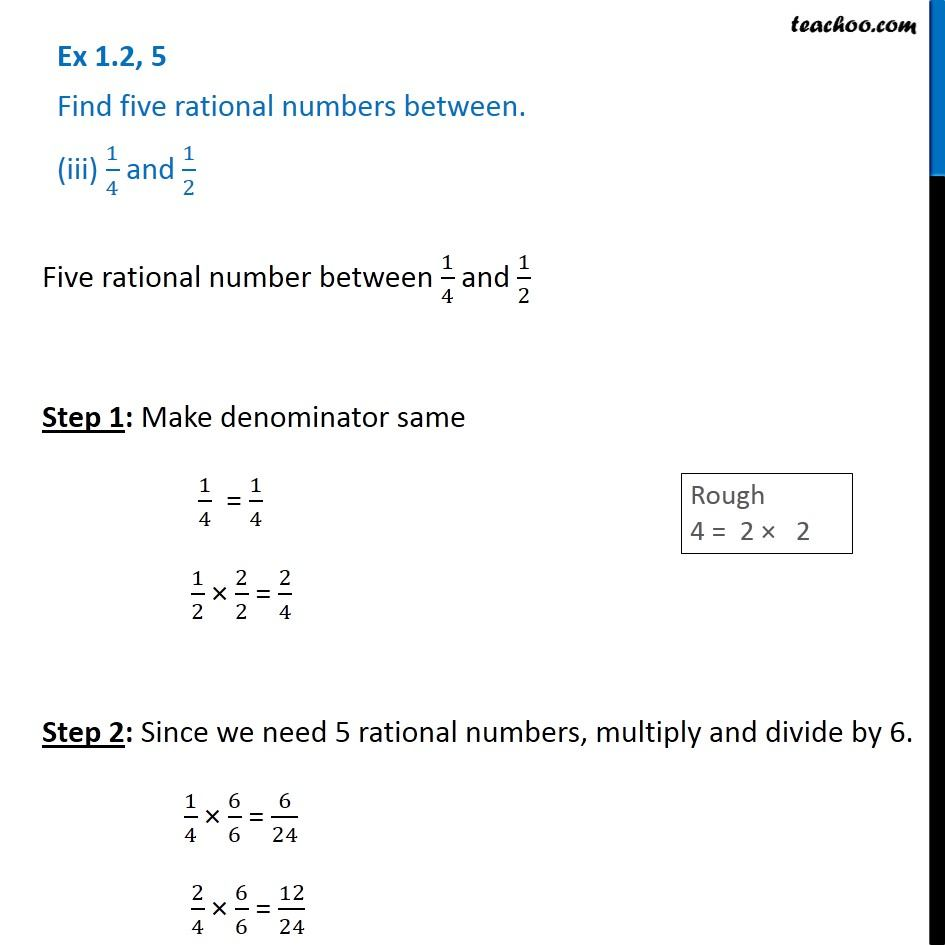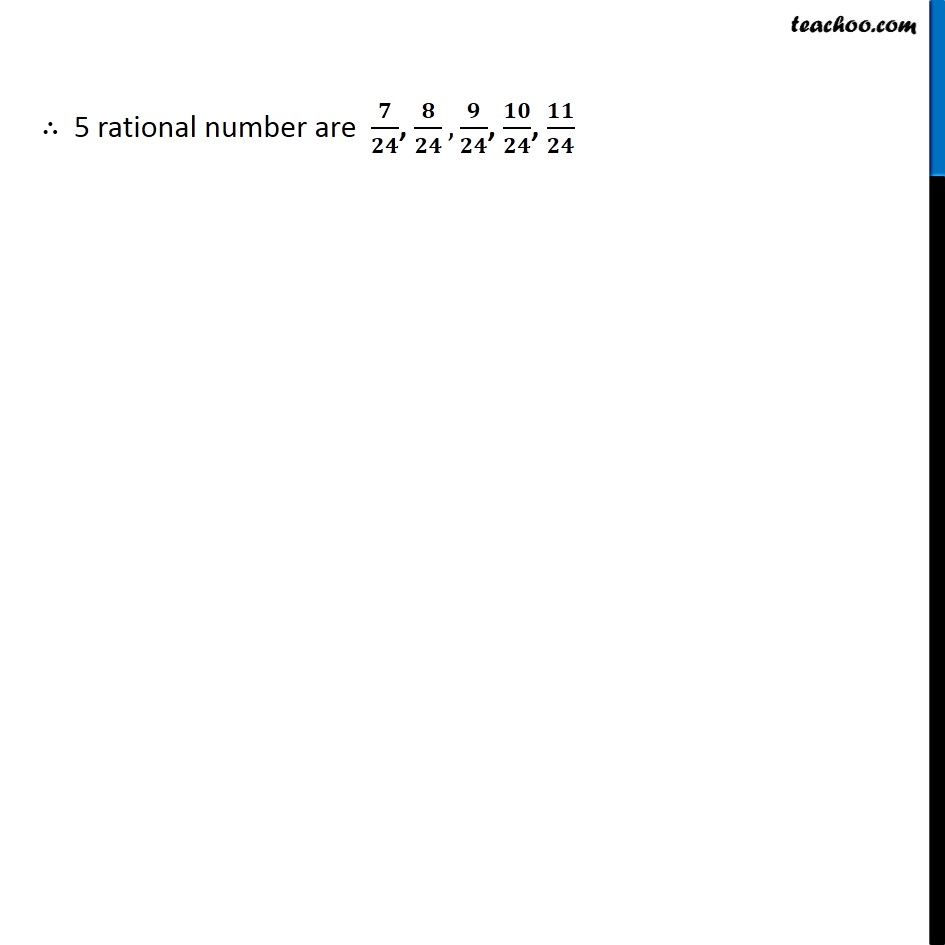Representing rational numbers on number line

Chapter 1 Class 8 Rational Numbers
Serial order wiseLearn in your speed, with individual attention - Teachoo Maths 1-on-1 Class

### Transcript

Question 5 Find five rational numbers between. (iii) 1/4 and 1/2 Five rational number between 1/4 and 1/2 Step 1: Make denominator same 1/4 = 1/4 1/2 × 2/2 = 2/4 Step 2: Since we need 5 rational numbers, multiply and divide by 6. 1/4 × 6/6 = 6/24 2/4 × 6/6 = 12/24 ∴ 5 rational number are 𝟕/𝟐𝟒, 𝟖/𝟐𝟒,𝟗/𝟐𝟒, 𝟏𝟎/𝟐𝟒, 𝟏𝟏/𝟐𝟒